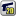﻿ Unreal Software - Thread: Bugs in "tibia".

# ForumCS2DScriptsBugs in "tibia".

#Bugs in "tibia".

3 replies
Goto Page1Melve
User
OfflineHi guys so i have a question to people who are creating their "tibia" or already created.

I had downloaded a "bug fixed" version but it doesnt work. I can still shoot monsters through the wall or get more attack, deff, speed, etc by dressing my items in spectator mode.

The question is somebody know how to fix it? I was trying for like 3 hours and still dont know how to do this.

Mora_rus
User
OfflineIs about monstersYou can rather use imagehitzone to these. I've made an monsters.lua for my roleplay but i dont care very much about it. So if you enough experienced of lua i could give you that and so you would take the parts and follow the way i did it.
Is not related to tibia so you cant just swipe files, you'll have to re-write it(but it based on weiwen's).
PM me if you're interested. Would be better to have discord contact..
TrialAndError
User
OfflineFor the Monsters:

Open 'functions.lua' and add these to the bottom:
Code:
1
2
3
4
5
6
7
8
9
10
11
12
function distance(x1, y1, x2, y2)
return math.floor(math.sqrt(math.pow(x1 - x2, 2) + math.pow(y1 - y2, 2)))
end

function freeline(x1, y1, x2, y2)
for i=1, distance(x1, y1, x2, y2) do
if not tile(math.floor((x1 + i * math.cos(math.atan2(y2 - y1, x2 - x1))) / 32),math.floor((y1 + i * math.sin(math.atan2(y2 - y1, x2 - x1))) / 32), "walkable") then
return false
end
end
return true
end

After you have added that, you can open up 'monsters.lua'.

Goto the function: 'MONSTERattack'.
Under
local rot = player(id, 'rot')
, you should see an if statement. Replace that with this:

Code:
1
2
3
if math.abs(math.rad(rot) - math.atan2(y-m.y, x-m.x) + math.pi/2)%(2*math.pi) <= (CONFIG.WEAPONWIDTH[weapon] or CONFIG.WEAPONRANGE) and freeline(player(id,"x"),player(id,"y"), m.x, m.y) then
closest = {m, dist}
end

There, we used the freeline function to check the tiles between the attacker and the monster. If it detects a tile that is not walkable, it will just return false and ignore the action.

For the Items:

For this one, goto 'hooks.lua'

Find the functions: 'EXPmenu' and 'EXPserveraction'
Right below where the functions starts, you should be able to an if statement,
if player(id, 'health') < 0 then return end

Replace the '<' with '<=' or just '==' as health don't get negative in CS2D (As long as I know, and if it does, it just gets reset to 0 anyways).
AbAeterno
User
Offline@TrialAndError: Just nitpicking here, but you could optimize the atan2 call by using a local variable instead of calling it twice (it doesn't sound like a function you want to be running way too often):
Code:
1
2
3
4
5
6
7
8
9
10
11
12
13
function distance(x1, y1, x2, y2)
return math.floor(math.sqrt(math.pow(x1 - x2, 2) + math.pow(y1 - y2, 2)))
end

function freeline(x1, y1, x2, y2)
for i=1, distance(x1, y1, x2, y2) do
local atan_ang = math.atan2(y2 - y1, x2 - x1)
if not tile(math.floor((x1 + i * math.cos(atan_ang)) / 32),math.floor((y1 + i * math.sin(atan_ang)) / 32), "walkable") then
return false
end
end
return true
end1﻿